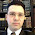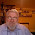## Tuesday, 8 February 2011

### E = mc2

There’s an entry in the Oxford BBC Guide to Pronunciation that got me thinking.It’s true — the default stress pattern in an algebraic expression involving a simple raising to the power of two is to deaccent squared.

The theorem of Pythagoras, which you will remember from school trigonometry classes, states (in the wording I was taught) that in a right-angled triangle the square on the hypotenuse is equal to the sum of the squares on the other two sides. Here, square and squares are regularly accented.
The ˈsquare on the hyˈpotenuse | is equal to the ˈsum of the ˈsquares on the ˈother two ˈsides.
Expressed as an algebraic equation, this takes the form
a2 + b2 = c2
which we say aloud as
ˈA squared plus ˈB squared | equals ˈC squared.

The word squared is not accented (unless of course it is contrastive). You might suppose that this is because we avoid accenting any item that is repeated or about to be repeated — a well-established principle in English accentuation.

However, this carries over, rather mysteriously, into cases where no repetition is involved.
ax2 + bx + c = 0
is said aloud as
ˈA ˈX squared | plus ˈ B X plus ˈC | equals ˈnought

Hence the constant in Einstein’s equation, the square of the speed of light, c2, has the spoken (recommended) default pattern ˈC squared.

So far, so good. But as soon as the item to be raised to the power of two is complex, involving more than one symbol, the word squared defaults to being accented. The expression (a + b)2 is said aloud as ˈA plus ˈB ˈsquared The expression (ax)2 is read as ˈA ˈX ˈsquared. I’m not sure why.

Hence the default interpretation of spoken ˈA ˈX squared is ax2, while the default interpretation of spoken ˈA X ˈsquared is (ax)2.

But a further way of avoiding the possible ambiguity in the latter is to say A X all squared. And in that wording you place the main accent on all — I think. Similarly, for (a + b)2 we can say ˈA plus ˈB all ˈsquared. Do you agree? It’s a long time since I did algebra.

None of this applies to cubed or higher powers. We say x3 as ˈX ˈcubed and x4 as ˈX to the ˈfourth.

1.ˈA ˈX squared | plus ˈ B x plus ˈC | equals ˈnought

Is the x in bx intentionally unaccented?

2.Algebraic expressions such as (ax)² are unusual IME because such an algebraic quantity would usually be rendered a²x². If someone needed to say (ax)² (let's say in the context of an algebra which is not known to be commutative, so it is not known that (ax)² and a²x² have the same value), I think it'd be prudent to say "a x all squared", because "a x squared" is liable to be understood as "ax²" even if the speaker tries to use stress and timing to suggest the contrary.

3.Give me time to get all the markup exactly right!

Yes, that X tends to undergo deaccentuation because of the operation of the 'rule of three' (English Intonation, p. 229).

4.Richard Sabey: you're right, and that is why (a+b)² vs a+b² is a better example.

5.Thanks, makes sense.

6.Both intonation and timing are important in the interpretation of such utterings, aren't they? 'A plus 'B squared is unambiguous to me, as is A plus B (mini-pause) 'squared. A plus B 'squared, not so much.

7.I think I've always accented ‘squared’ in πr². Or maybe all three elements are equally accented.

8.For ax² + bx + c = 0, I would say ˈA X SQUARED | plus ˈ B X plus ˈC | equals NOUGHT. (I've used caps instead of underlining because I can't work out how to underline.)

That is, the same as what John's got above, but with the squared heavily accented. This is because you're contrasting x² with x - that is, X squared with X.

I'd suggest the reason E = m is accented the way it is is because it's most commonly heard spoken by non-specialists: laymen who may not understand the formula. The other examples are less well known and likely to be heard by GCSE maths teachers, schoolchildren and other people who (are supposed to) understand its meaning.

9.Should we not make a distinction here between speech as a sole mode of communication and speech as an adjunct to visual symbolisation?

If I needed to dictate (a + b)2 over the phone, I would say something like a plus b in brackets all squared.

When we heard a teacher say these things in a classroom, we could often see the precise formulation on the board or in our books. If not, the chances were that we had just been looking and hearing about something very similar.

The point about E = mc2 is that we all know what the equation is, but few of us know what it means.

As it happens, I do 'understand' in the sense that I know what each symbol represents and which of them are multiplied and which squared. This is necessary but insufficient, since I barely understand what a physicist means by mass and energy and don't understand at all how they are quantified in this equation.

The wording of the equation is a cultural allusion that I do understand. I might be a little thrown if I heard it with different stress.

10.@Steve Doerr:
Indeed the pun reply "No, pie are not square, they are round; cornbread are square" doesn't work if the ^2 is unstressed. Neither does TSTN^2 for "there's something that's not square".

(Why is the <sup> tag not allowed?)

11.You can use &sup2; if you want a superscript 2.

12.Steve

Thanks for that. I copied a superscript from the OP but it progressively lost effect after I pasted.

13.I feel the authors of the Oxford BBC Guide to Pronunciation have got themselves all fussed over a non-problem, because, as Richard Sabey explained above, the priority rules, both in writing and in speech, avoid the supposed ambiguity. I don't think anyone who cared about what the equation means would ever take it to mean that both the m and the c were squared, even when meeting it for the first time. Moreover, while fussing over a non-problem the authors have introduced a worse one, writing mc2 (without italics, and with no superscript. On the Internet that is excusable, but you example appears to be printed, and in print there is no excuse, especially from people who can print phonetic characters.

14.csquared m would work. There's no particular reason to have the m first. But, as has been pointed out, the traditional order is more of a saying than a formula. It's been part of popular culture for so long that it would only seem pedantic to use another form

15."None of this applies to cubed or higher powers."

Well, no. I disagree. You just haven't gone through the parallel set of examples. Like (a + b)3, for example (forgive me for not attempting the markup here). Accentuation would be exactly the same as for (a + b)2.

16.I don't agree with much of the above description. For instance, I would say "'E equals 'M C 'squared." For quadratic equations, I say "'A X 'squared ..." I've never found it odd among my peers. Is this an American thing?

17.I agree with c.c. and Pete and Steve. I don't use, and have never heard, a deaccented 'squared' in these examples.

18.@ c.c.: I say those the same way you do. That's how I've always heard them pronounced in the Midwest. Maybe that's a common pronunciation in the U.K. too and that's why the BBC Guide to Pronunciation has to tell people not to pronounce it that way.

19."But a further way of avoiding the possible ambiguity in the latter is to say A X all squared. And in that wording you place the main accent on all — I think."

I may well be wrong, but I don't recall ever hearing this variant through ten-odd years at uni. So I wouldn't naturally use it myself when teaching either.

I agree with Luke that the stress seems to follow from making a short pause before "squared" so as to 'pronounce' the parenthesis. But it's ambiguous, yes, and I usually get questions for clarification, yes. I tend to draw the parentheses in the air much like airquotes, when I'm not writing on the board.

20.@Anonymous, @c.c.:

I think that the normal pronunciation in the UK also has stress on "squared". The BBC guide is being prescriptive here, not descriptive: it explicitly admits that "the formula is often pronounced with stress on the m and the squared rather than the c".

21.Familiarity is an important factor here. If the function were ax^3 + bx^2 + cx + d, rather than a quadratric with ax^2 etc, then the exponents would have to be stressed because it is not such a common function.
in forms such as (a+b)^n, I would use 'all to the n' by default, but I'm wondering when i mightn't put it in. Having something multiplied before the bracket seems to matter:
so, (perhaps)
(a+b)^n is 'a plus b all to the n', but
p(a+b)^n is 'p times a plus b (pause) to the n'

22.Many mathematicians pronounce (a + b)² as "a plus b quantity squared", where the word "quantity" means "do the arithmetic I've described so far before proceeding. So we would say, for instance "X plus Y quantity squared equals X squared plus 2 X Y plus Y squared" to convey the identity (X + Y)² = X² + 2XY + Y².